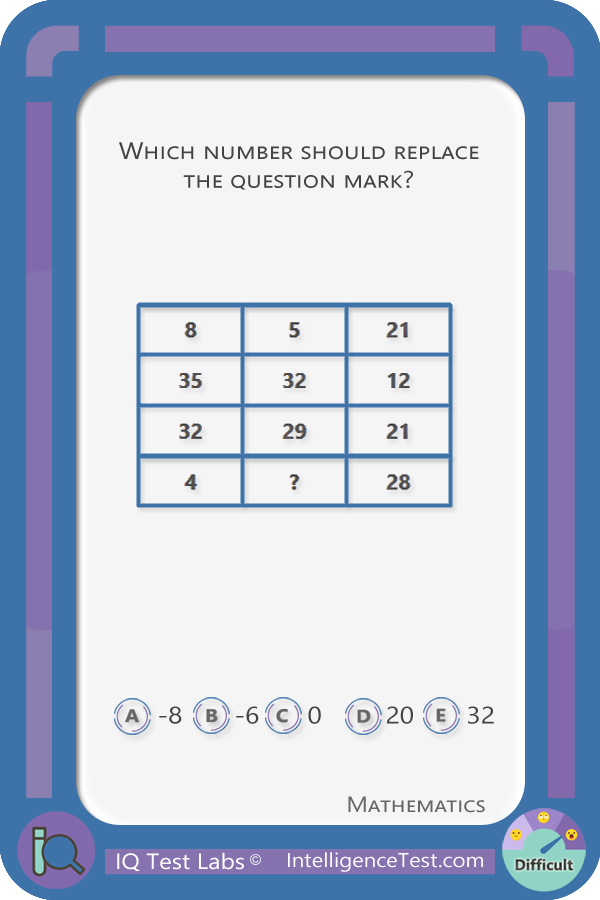# IQ Test Labs

## 2/10 Number matrixFor each row, the third column involved calculating the sum of the digits.

* Required information
1000
What is ten divided by two?New
Katariinasays...

I answered zero, because if you subtract the two digits that are in the same box, you'll get:

8, 5, 1

-2, 1, -1

4, ? , -6

Then if you add the rows you get: 13 (8+5+1), -2 and -6. I you add them up, it becomes zero, so the whole thing to keep the answer as zero, the ? should be zero. Possible solution, but wrong here.New
Aaronsays...

First row: 8,5,21= 21 (2+1)=3. 8-3=5

Second row 35,32,12= 12 (1+2)=3. 35-3=32

Third row 32,29,21= 21(2+1)=3. 32-3=29

Fourth row 4,?,28=28 (2+8)=10. 4-10=-6 (the answer)New
Khaledsays...

i only guessed it right and do not no why -6 is the answerNew
massays...

For each row subtract the second column from the first column. The result is equal to the sum of the digits in the last column.New

This is the simple explanation

8-5=3 21/3=7

35-32=3 12/3=4

32-29=8 21/3=7

4+6=10 7+7+3+10=28

### widgets Latest post

#### Get new questions to hone your IQ!### whatshotIn the news

#### Keeping the beat### pie_chartPolls

#### Quotations